# Astronomy 12 - Spring 1999 (S.T. Myers)

## Problem Set #4 (due Thu 4 Mar 1999 5pm)

### Last Revised 3 Mar 1999

1. An O8 main sequence star has a luminosity of 170000 L_sun and a mass of 23 M_sun. If the Sun has a lifetime of 10 billion years on the main sequence, approximately how long will the O8 star last?

An M8 dwarf star, one of the wimpiest main sequence stars known, has a luminosity of only 0.0012 L_sun and a mass of 0.06 M_sun. What would its lifetime be?

If the O8 star has an effective temperature of 35000 K, and the M8 of 2600 K , estimate their radii (in R_sun).

Estimate the central pressures and temperatures for these stars.

2. Consider a large 10^4 M_sun molecular cloud with a H_2 density of n_H2 = 5 x 10^9 m^-3, at a temperature of 200 K. Inside this cloud, there is a dense core with a mass of 5 M_sun at a temperature of 10K. Assuming that the core is uniform in density, spherical in shape, and is in pressure equilibrium with the cloud, find the density in the core (in m^-3) and the radius of the core (in pc).

The core will collapse if the the total gravitational energy is greater than the thermal energy

G M2 / R   >   M kT / µ m_H

where the mean molecular mass of H_2 is µ = 2. Will this core collapse?

For our 10 K cloud core with the density determined above, what is this minimum mass (in M_sun) for collapse?

Assuming that it can collapse no faster than the free-fall time, what is the minimum number of years it will take to collapse (assume it collapses effectively all the way to a point for this calculation)?

3. Is there a limit on how luminous a star can be? Consider a very luminous star. In its photosphere, the pressure gradient will be dominated by radiation pressure

Prad = 4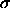/3c T4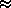Frad / c

(which is the momentum transfered through the photospheric layer) where F_rad is the radiative flux. Using the radiative transfer equation

dF = -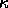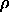F dr

dPrad / dr = -/c L / 4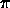r2.

If this radiation pressure gradient is higher than the limit for hydrostatic equilibrium

dP/dr = - GM/ r2

then the photosphere will be blown away by the radiation pressure! Show that the maximum luminosity that a star can sustain is given by

LEd = 4GMc /which is also known as the Eddington luminosity, after the great astrophysicist Sir Arthur Eddington. This luminosity limit appears in a number of areas of astrophysics, including late stages of stellar evolution, novae, quasars, and accretion disks.

In massive, luminous stars, the photospheric opacity will be dominated by electron scattering=T / mH0.04 m2/kg.

What is the Eddington luminosity for a 90 M_sun star at the upper end of the main sequence?

4. The atomic mass unit is defined as exactly 1/12 the mass of the Carbon-12 nucleus:

1 amu = 1.660540 x 10-27 kg.

The mass of a hydrogen nucleus is 1.007825 amu and the mass of helium-4 is 4.002603 amu, and of course carbon-12 is 12 amu.

Compute the mass difference between four hydrogen nuclei (protons) and a single helium-4 nucleus in amu and in kg. What fraction of the four proton masses is this?

Convert this mass difference (called the mass defect into an equivalent energy using

E = m c2

and express this energy in both Joules and electron volts (this is equal to the binding energy of the helium nucleus). This is the energy released each second in a single fusion reaction (pp or CNO cycle). How many fusion must occur each second to account for the solar luminosity?

Assume that 13% of the Sun's mass is in its core (and that 75% if it is hydrogen), and is available for fusion over its lifetime. How long could the Sun last at a constant luminosity equal to its current luminosity with this amount of fuel?

In the triple-alpha reaction, helium is burned into carbon. Calculate the energy (in Joules) released in the fusion of one kg of helium into carbon.

When our Sun reaches the red giant stage and starts to burn helium in its core, it will have a luminosity of around 100 L_sun with about 13% of its mass in the helium core. How many years will the Sun be able to sustain helium burning at this luminosity before running out of fuel?

When a star is buring helium, it is in a part of the H-R diagram called the horizontal branch, and is a supergiant. Comparing the relative lifetimes of a solar-mass on the hydrogen-burning main sequence and the helium-burning horizontal brance, estimate the relative numbers of stars that you should find in these respective parts of the H-R diagram. (Hint: Just calculate the ratio of the times burning hydrogen and burning helium, thats all Im asking.)

5. A main-sequence star of spectral type F0 V is expected to have an absolute visual magnitude M_v = +2.6 and a color B-V = +0.30. A star that is expected from its spectrum to be of this type is actually observed to have an apparent magnitude of m_v = 19.1, and a color of B-V = +0.8. What is the distance to the star, the implied dust extinction A_v in magnitudes, color-excess CE in magnitudes, and dust optical depth along the line of sight to this star? (Remark: the parallax given previously in this problem was inconsistent, and should not be used! Sorry about that...)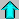Astr12 Index ---Astr12 Home

smyers@nrao.edu Steven T. Myers